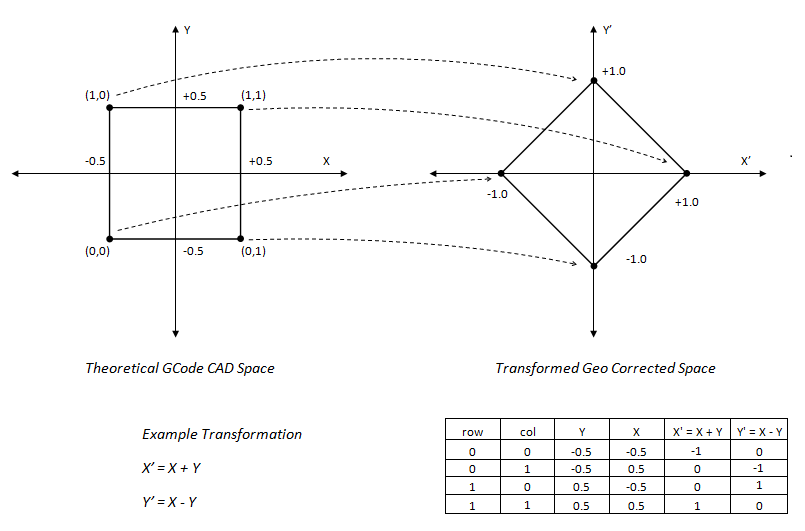# Geo Correction Table

The KMotion Coordinated Motion/Trajectory Planner/Kinematics Libraries contain a powerful Geometric Correction Capability. A Geometric correction table can be used to correct for Geometric distortion from the Theoretical CAD Space to the Corrected Actuator space.The area of the CAD Space to be corrected is defined by a number of rows and columns and an XY Grid size. A CAD point falling within a grid uses a bilinear interpolation from the 4 points making up the grid region to map to actuator space.

There is also a Z coordinate associated with each grid point. The mapping applies this as a Z offset in order to flatten the XY plane if necessary. If no flattening is required then the z values should all be specified as zero in the table.

Normally a Geo Correction Table is defined to be large enough to map the entire range of motion of the system. However, for points outside the range of the Table the closest grid region is extrapolated to form the result. The largest Table currently allowed is a 4000x4000 array.

When a Geo Correction Table has been loaded Arcs are automatically broken into small line segments internally where each segment's endpoints are Geocorrected into actuator space. This is necessary because an Arc in CAD space in unlikely to still be an Arc in Actuator Space. The Trajectory Planner Collinear Tolerance Setting is used to determine how small of line segments are used. Line segment lengths will be chosen small enough to not deviate from the true Arc by more than the Collinear Tolerance. For most systems a value of 0.001 inches should be used.

The smallest possible Geo Table consists of a single rectangle consisting of 4 points (2 rows and 2 columns). In this case the entire infinite CAD Space plane is mapped to the Actuator Space using a single set of transformation equations.

The Example below shows how the table can be used to form a simple transformation:Corresponding Geo File:

Simple 2x2 grid on CAD Space Grid Spacing of 1 inch in X and 1 inch in Y.

Then 4 grid points (row,col,X,Y,Z)

See file as <Install>\KMotion\Data\MeasurementsPlusMinus.txt

2,2
1,1
-0.5,-0.5
0,0,-1, 0, 0
0,1, 0,-1, 0
1,0, 0, 1, 0
1,1, 1, 0, 0